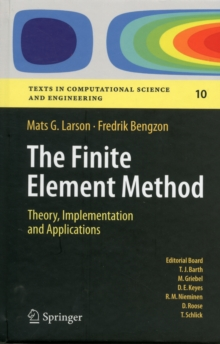Supporting your high street Find out how »
• My Account# The Finite Element Method: Theory, Implementation, and Applications Hardback

## Part of the Texts in Computational Science and Engineering series

#### Description

This book gives an introduction to the finite element method as a general computational method for solving partial differential equations approximately.

Our approach is mathematical in nature with a strong focus on the underlying mathematical principles, such as approximation properties of piecewise polynomial spaces, and variational formulations of partial differential equations, but with a minimum level of advanced mathematical machinery from functional analysis and partial differential equations.

In principle, the material should be accessible to students with only knowledge of calculus of several variables, basic partial differential equations, and linear algebra, as the necessary concepts from more advanced analysis are introduced when needed.

Throughout the text we emphasize implementation of the involved algorithms, and have therefore mixed mathematical theory with concrete computer code using the numerical software MATLAB is and its PDE-Toolbox.

We have also had the ambition to cover some of the most important applications of finite elements and the basic finite element methods developed for those applications, including diffusion and transport phenomena, solid and fluid mechanics, and also electromagnetics.

#### Information

• Format: Hardback
• Pages: 395 pages, XVII, 395 p.
• Publisher: Springer-Verlag Berlin and Heidelberg GmbH & Co. K
• Publication Date:
• Category: Differential calculus & equations
• ISBN: 9783642332869

#### Other Formats

£64.99

£53.75

on all orders

###### Pick up orders

from local bookshops

£53.99

£45.89

£53.99

£45.05

£54.99

£45.85

£55.00

£46.75

£44.99

£36.95

£49.99

£40.79